# On hidden realities (part 3)

This is our third part of a series of posts on (a few selected) hidden realities : many-worlds interpretation of quantum mechanics, multiverse linked to the space-time geometries and dynamics, higher dimensional multiverse, holographic multiverse and simulated multiverse.

For the previous posts, please see:

This time, we’ll enter the world of higher dimensional theories.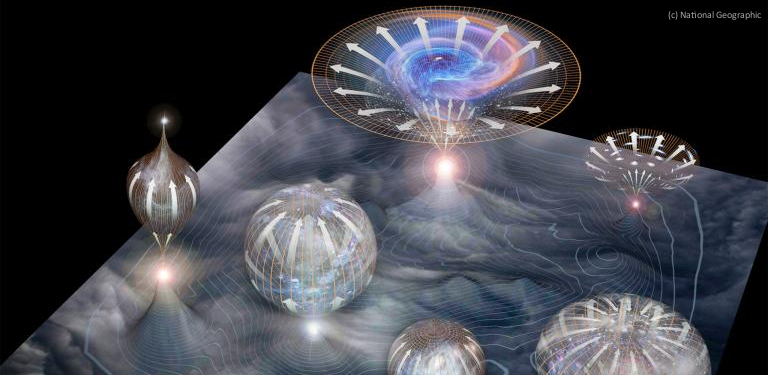Electromagnetism

Electromagnetism is the study of the electromagnetic force which is the physical interaction that occurs between electrically charged particles. The electromagnetic force is one of the four fundamental interactions, the ones being the strong interaction, the weak interaction, and gravitation.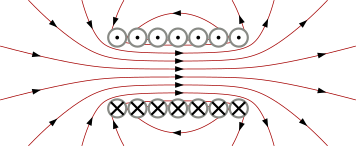If a particle of charge q moves with velocity v in the presence of an electric field E and a magnetic field B, then it will experience a force called Lorentz force:$\mathbf{F}=q[\mathbf{E}+(\mathbf{v}\times \mathbf{B})]$

Electromagnetism theory, as John Keats would have said, is a thing a beauty, a joy forever:

Indeed, Maxwell equations are unifying the electric and magnetic fields into a set of partial differential equations (in Planck’s units):In relativistic terms, four-vector notation are used. Using a (+,-,-,-,-) signature for Minkowski metric tensor, one can introduce these notations:

• 4-position:$x^\mu = (t, x, y, z)$
• 4-momentum:$p^\mu = (E, \mathbf p)$
• 4-gradient:$\partial_\mu = (\partial / \partial t, \nabla)$
• 4-current:$J^\mu = (\rho, \mathbf J)$
• 4-potential:$A^\mu = (\phi, \mathbf A)$

With these, the electromagnetic tensor (also called Faraday tensor) can be written down as:$F_{\mu\nu} = \partial_\mu A_\nu - \partial_\nu A_\mu$

It is a covariant representation of the electromagnetic field. In these terms, Maxwell equation simplifies beautifully as:$\partial_\mu F^{\mu\nu} = J^\nu$

Geometrically speaking, the electromagnetic tensor is … a curvature and electromagnetism is a connection on a (circle) U(1) bundle. Indeed, we’ve already seen that quantum electrodynamics can be expressed abelian gauge theory with the symmetry group U(1). The simplest relativistic Lagrangian invariant under local U(1) transformation can be written in terms of Faraday tensor :$\mathcal{L}_{QED} = i \bar{\psi}\gamma^{\mu}\partial_{\mu}\psi - e \bar{\psi} \gamma_{\mu} (A^{\mu} + B^{\mu})\psi - m \bar{\psi} \psi - \frac{1}{4} F_{\mu\nu} F^{\mu\nu}$

Which is simply amazing ! Electromagnetism, conceived in the 1800’s, is a relativistic unified theory that one can also turn into a beautiful quantum theory.

Kaluza-Klein theory: adding a 5th dimension

Let’s get back to the early 1920’s. At the time, only two fundamental interactions were known: gravitation and electromagnetism, the latter being a theory unifying electrical and magnetic interactions. It was on logical to try to unify gravitation and electromagnetism as James Clerk Maxwell did 40 years ago at the time.

Theodor Kaluza took Einstein’s general theory (which was brand new) and extended it to five dimensions. The result is quite extraordinary:

• Part of Kaluza’s result can be decomposed into … Einstein field equations in a classical 4 dimensional space-time
• Part of Kaluza’s result can be identified to … Maxwell field equation for electromagnetism
• The rest can be identified to a Klein-Gordon equation, introducing a new scalar field which quantum as called “radion” (or sometimes “dilaton”)

This a stunning result. But it has (at least) one drawback: what to do with this 5th dimension that nobody has ever seen ?

In 1926, Oskar Klein (the physicist, not the other Klein who’s bottle is so famous) dipped Kaluza’s theory into quantum physics. He’s idea was to curl this new dimension up to microscopic scale. This idea is nowadays called compactification.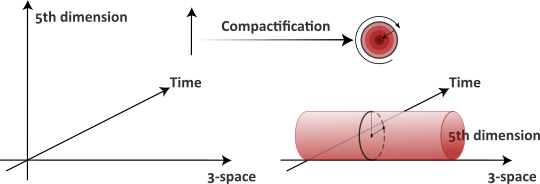The 5th dimension (which would be a 4th space-like dimension) is curled up in a circle of an extremely small radius (10-35 m): in every point of space time there is one extra dimension rolled-up into a circle.

In modern geometry, this extra dimension is understood as a circle group U(1), precisely enable theorist to formulated a gauge theory on a fiber bundle with gauge group U(1). One sees how it could be generalized to the SU(3) x SU(2) x U(1) symmetry group of the Standard Model of particle physics and include the other fundamental interactions.

But, it turns out that Kaluza-Klein is flawed… For example, it leads to a calculated mass of the electron many orders of magnitude greater than the measured one. Too bad.

String theories and M-theory: 10, 11, 26 dimensions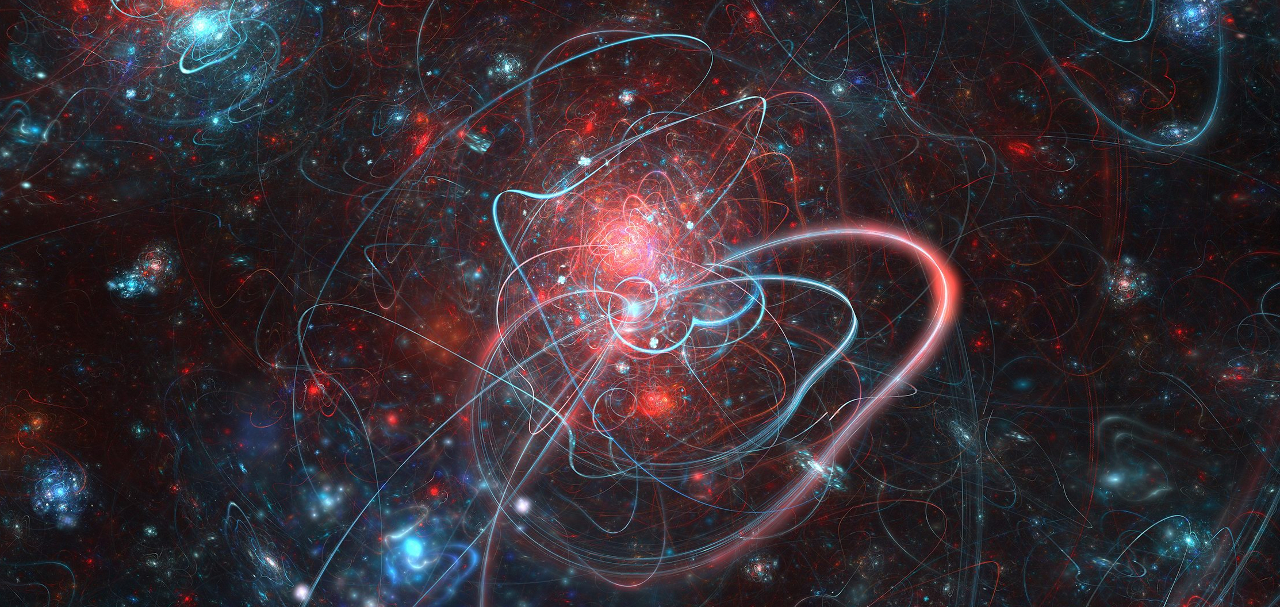Even if Kaluza-Klein theory turned somewhat sour, higher dimensions and compactification turned out to be seeds of new theories.

Contemporary physics theories often need higher dimensions to be mathematically consistent :

Somehow, the natural progression was to add more dimensions and see what happens when it is compactified.

But there are many many ways to compactify extra dimensions, and herein lies the problem: nobody knows just what shape it should be, and different physics come from differents shapes. It is usually assumed that extra dimensions are “wrapped” up on themselves, or “curled” up on a 6-dimensional Calabi–Yau space on each point of space: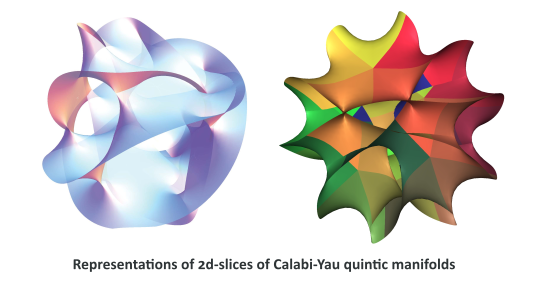If the compactification possibilities have been narrowed down to this type of shape, it still leaves a huge (possibly infinite) number of specific shapes.

Even though there theories are technically quite different from Kaluza and Klein’s , the coupling constant of string theory, which determines the probability of strings to split and reconnect, can be described by a field called … dilaton.

Moreover, physicists have speculated that the graviton, the particle carrying the force of gravity, may “leak” into the fifth or higher dimensions, which would explain how gravity is significantly weaker than the other three fundamental interactions. This is a beautiful idea. My subjective opinion is that string theories (as they stand now) are more exquisite mathematics than real physics. But some of its ideas (and mathematics) are probably the seeds of news fruitful theories.

Experimentation will tell.

Alternative interpretation of extra dimensions: the holographic principle

In 1993, Gerard ‘t Hooft put forward the holographic principle, which explains that the “information about an extra dimension is visible as a curvature in a space-time with one fewer dimension”.

Familiar holograms are three-dimensional pictures placed on a two-dimensional surface, which gives the image a curvature when the observer moves.

Similarly, in general relativity, the fourth dimension is manifested in observable three dimensions as the curvature path of a moving infinitesimal. ‘t Hooft has even speculated that the fifth dimension is really the space-time fabric. We will speak a bit more of the holographic principle on our next post.

Poetic ending

For once, let’s forget films, and let’s talk literature.

“Flatland: A Romance of Many Dimensions” is an 1884 book written by Edwin Abbott Abbott. The author uses a fictional two-dimensional world baptized Flatland to satirically comment on the hierarchy of Victorian culture.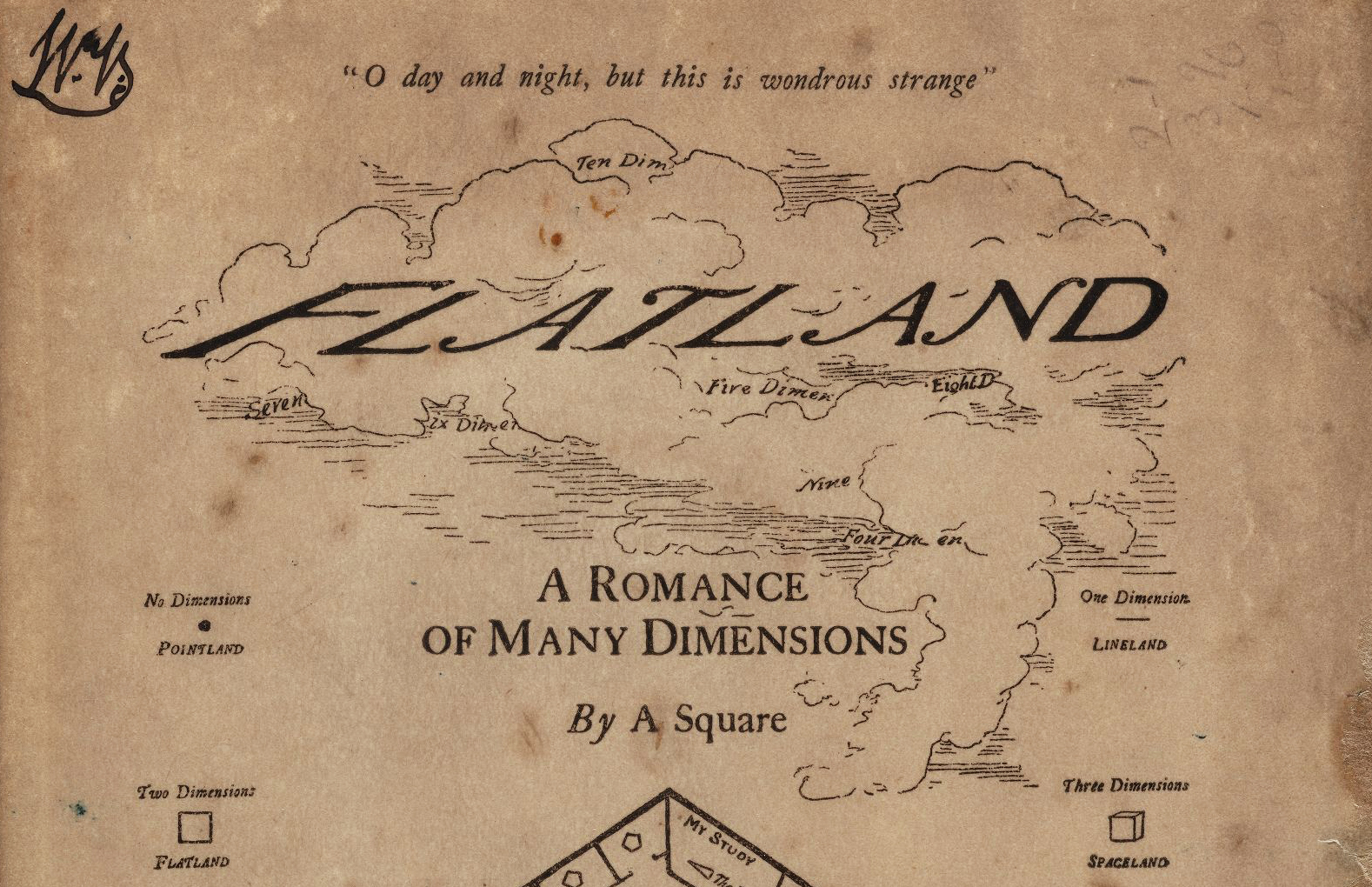At the time of its publication, tbe book did not attract as much attention as it deserved. The book was later discovered again, after Albert Einstein’s general relativity was popularized, introducing the concept of a fourth dimension to all :

If there is motion of our three-dimensional space relative to the fourth dimension, all the changes we experience and assign to the flow of time will be due simply to this movement, the whole of the future as well as the past always existing in the fourth dimension.

—from a “Letter to the Editor” by William Garnett. In Nature on February 12, 1920.

Let’s quote the book:

The diminished brightness of your eye indicates incredulity. But now prepare to receive proof positive of the truth of my assertions. You cannot indeed see more than one of my sections, or Circles, at a time; for you have no power to raise your eye out of the plane of Flatland; but you can at least see that, as I rise in Space, so my sections become smaller. See now, I will rise; and the effect upon your eye will be that my Circle will become smaller and smaller till it dwindles to a point and finally vanishes.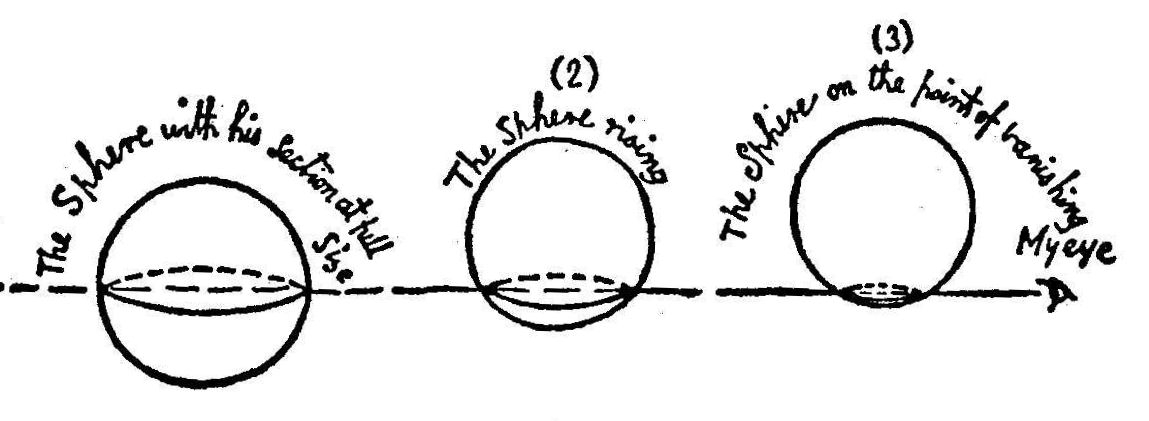There was no “rising” that I could see; but he diminished and finally vanished. I winked once or twice to make sure that I was not dreaming. But it was no dream. For from the depths of nowhere came forth a hollow voice – close to my heart it seemed – “Am I quite gone? Are you convinced now? Well, now I will gradually return to Flatland and you shall see my section become larger and larger.”

In 2001, Ian Stewart, renowned popular-science writer, published “FlatterLand: Like Flatland, only more so” as a sequel to “Flatland”. It is a travel in the “Mathiverse”, a set of all imaginable worlds, visiting higher dimensions modern theories, and ending the “Domain of the Hawk King” to discuss time travel and the theory of relativity.

## 2 thoughts on “On hidden realities (part 3)”

•Samuel S.Y. Wong says:

Dear Dr. Frédéric Bellaiche,
I am an undergraduate physics researcher working on the physics of compactified space. I would like to ask for permission for modifying and using your “5th dimension” image in a powerpoint presentation at a conference. Would you let me do that?

Thank you very much,
Samuel S.Y. Wong

•Fred Bellaiche says:

Hi,

Yes, please do. No problem whatsoever 🙂

This site uses Akismet to reduce spam. Learn how your comment data is processed.